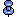# Statistical types

The statistic type defines the duration of the data item (sample or session) and whether the server performs calculations on the data item.

The six statistic types are:

• SMC_STAT_VALUE_SAMPLE – this statistic type returns a count of activity or some type of information that applies to the most recent sample interval. No calculations are performed.

• Activity counts – for data items that represent activity counts, SMC_STAT_VALUE_SAMPLE returns the number of occurrences of an activity during the most recent sample interval. For example, SMC_STAT_VALUE_SAMPLE for SMC_NAME_PAGE_IO is the number of page I/Os that occurred during the most recent sample interval.

• Other information – this is the only statistic type valid for data items that represent character strings. For example, SMC_STAT_VALUE_SAMPLE for SMC_NAME_OBJECT_NAME returns the name of a database object. This statistic type is also the only one valid for data items that represent values such as IDs and values for configured parameters, on which calculations are never performed.

• SMC_STAT_VALUE_SESSION – this statistic type returns a cumulative count of activity since the start of gathering the data (since the connection was opened). No calculations are performed. For example, SMC_STAT_VALUE_SESSION for SMC_NAME_PAGE_IO is the number of page I/Os that occurred since the session started.

• SMC_STAT_RATE_SAMPLE – this statistic type calculates a rate per second. It returns the average number of occurrences per second of an activity during the most recent sample interval. For example, SMC_STAT_RATE_SAMPLE for SMC_NAME_PAGE_IO is the average number of page I/Os that occurred each second during the most recent sample interval.

The calculation is count for the most recent sample interval divided by number of seconds in the sample interval.

• SMC_STAT_RATE_SESSION – this statistic type calculates a rate per second. It returns the average number of occurrences per second of an activity during the current session. For example, SMC_STAT_RATE_SESSION for SMC_NAME_PAGE_IO is the average number of page I/Os that occurred per second since the session started.

The calculation is count for the session divided by number of seconds in the session.

• SMC_STAT_AVG_SAMPLE – this statistic type calculates an average value per occurrence of an activity over the most recent sample interval. Only a few data items can use this statistic type. The meaning of the returned value depends on the data item name. For example, SMC_STAT_AVG_SAMPLE for SMC_NAME_STP_ELAPSED_TIME is the average execution time per execution of a stored procedure during the most recent sample interval.

• SMC_STAT_AVG_SESSION – this statistic type calculates an average value per occurrence of an activity over the session. Only a few data items can use this statistic type. The meaning of the returned value depends on the data item name. For example, SMC_STAT_AVG_SESSION for SMC_NAME_STP_ELAPSED_TIME is the average execution time per execution of a stored procedure during the recording session.Not all statistical types are valid for all data items. See Chapter 2, “Data Items and Statistical Types” for more information about data items and the rules for using them.